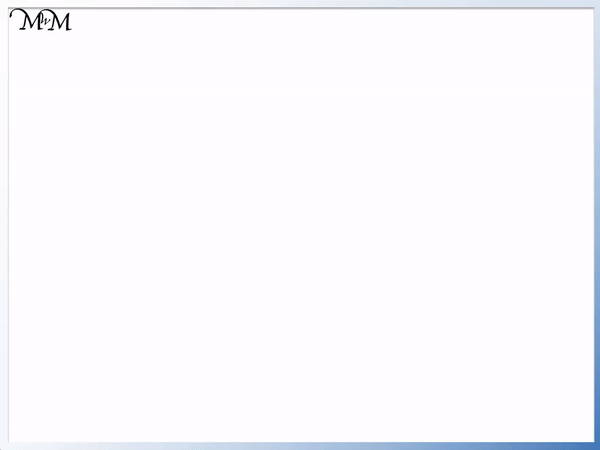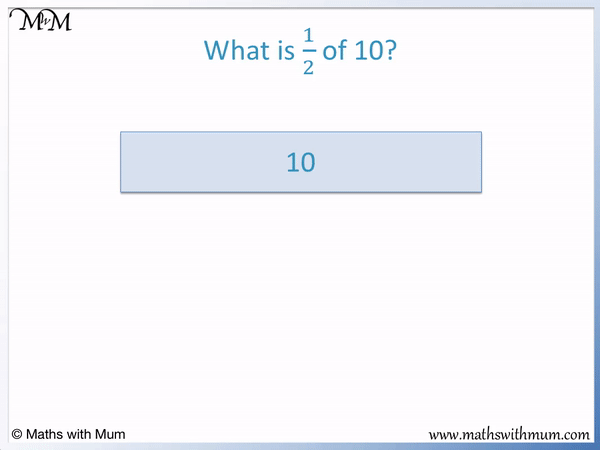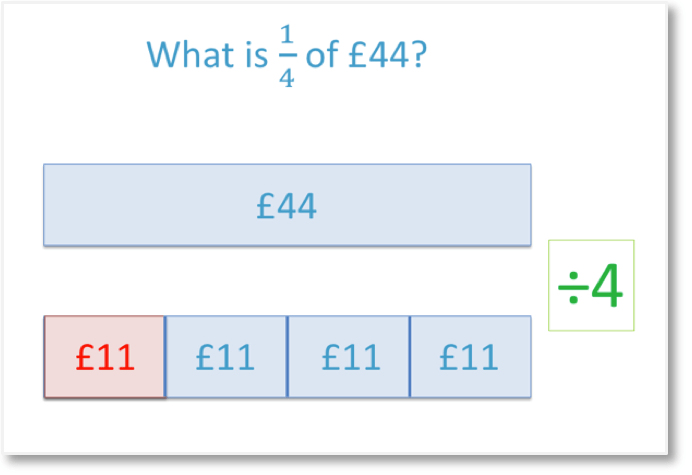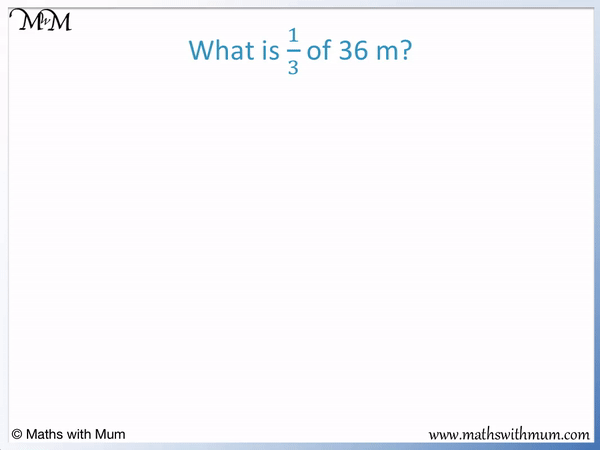# Unit Fractions of Amounts

Unit Fractions of Amounts• A unit fraction is a fraction that has a 1 on top.
• A unit fraction has a of 1.
• The on the bottom of the fraction can be any other whole number.
• To find a unit fraction of an amount, divide the amount by the denominator on the bottom of the fraction.
• The denominator tells us how many equal parts to divide our amount into.
• We divide 18 into three parts to find   1 / 3   of 18.
• 18 ÷ 3 = 6.
• One third of 18 is 6.
A unit fraction has a numerator of 1.

To find a unit fraction of an amount, divide the amount by the denominator.

#### Examples of Unit Fractions

Below are some examples of different unit fractions.• To calculate a unit fraction of an amount, divide the amount by the of the fraction.
• The denominator is the number on the bottom of our fraction, which is 5.
• 1 / 5   is said as 'one fifth'.
• To find one fifth of an amount, divide it by 5.
• 50 ÷ 5 = 10.
• Therefore,   1 / 5   of 50 is 10 grams.Supporting Lessons# Unit Fractions

## What are Unit Fractions?

A unit fraction is a fraction that has a numerator of 1. This means that the number on the top of a unit fraction must be a 1. The denominator on the bottom of a unit fraction can be any other whole number.

Unit fractions are called this because they have a numerator of 1. Unit means 1. The name of unit fraction tells us that this fraction must have a numerator that is equal to 1.

The first examples of unit fractions are:

Unit Fraction Name
1/2 One half
1/3 One third
1/4 One quarter
1/5 One fifth
1/6 One sixth

After the first few unit fractions shown above, the rest of the unit fractions are pronounced by saying "one" then the number then "th". For example,   1/10   is pronounced as "one tenth".

Here is a poster showing the size of unit fractions and pictures of unit fractions.Unit fractions always have a numerator of 1. The number on the bottom can be any other whole number.

The numerator of a fraction is the number on the top of it. The numerator tells us how many parts we have.

The denominator of a fraction is the number on the bottom of it. The denominator tells us how many parts there are in total.

One quarter is an example of a unit fraction because it has a 1 on top as its numerator.The denominator of one quarter is a 4, which means that the shape is divided into 4 parts.

We can see that the larger the denominator of a unit fraction, the more parts we have divided the amount into and the smaller the size of the fraction.

A unit fraction must have a numerator of 1. A fraction that is not a unit fraction is called a non-unit fraction. Non-unit fractions have a numerator that is larger than 1.

## How to Find Unit Fractions of Amounts

To find a unit fraction of an amount, divide the amount by the denominator of the fraction. For example to find   1/3   of 18, divide 18 by 3. 18 ÷ 3 = 6 and so, one third of 18 is 6.

1/3   is a unit fraction with a denominator of 3. To find   1/3   of 18, divide 18 by 3.Unit fractions can be represented using a bar model. The full bar represents the total amount and this can be divided up into equal parts to represent the unit fractions.

Here the whole bar is worth 18 and we can divide it into 3 equal parts to find one third.18 ÷ 3 = 6 and so,   1/3   of 18 = 6.

We can work this out by thinking, "What number do I need to multiply 3 by to get 18?"

3 × 6 = 18 and so one third of 18 is 6.

Here is another example of finding a unit fraction of a number.

In this problem we are asked to find   1/2   of 10.To find a unit fraction of a number, divide the number by the denominator of the fraction.

To find   1/2   of 10, divide 10 by 2.10 ÷ 2 = 5 and so, one half of 10 is 5.

Here is another example of calculating a unit fraction of an amount.

In this unit fraction problem, we have to find   1/4   of £44.If there are units in a unit fraction problem, simply ignore them, do the calculation and then put them back in at the end.

We divide by the denominator of the fraction. The denominator is 4.44 ÷ 4 = 11 and so, £44 ÷ 4 = £11.

One quarter of £44 is £11.

Here is an example of finding the unit fraction   1/3   of 36 m.

To find a unit fraction of an amount, divide by the denominator. The denominator of one third is 3. To find one third, divide by 3.36 ÷ 3 = 12 and so, 36 m ÷ 3 = 12 m. One third of 36 metres is 12 metres.Now try our lesson How to Find Fractions of Amounts, where we learn how to find non-unit fractions of amounts.error: Content is protected !!